Constructing shear force and bending moment diagrams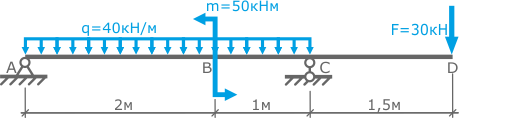For a given two-bearing beam loaded with a force F, a moment M and a uniformly distributed load q, plot the plots of the internal transverse forces Qy and the bending moments Mx.

The solution of the problem

The reference reactions for this design scheme were defined here.

The beam has 3 power sections. Denote them by Roman numerals, for example, from right to left.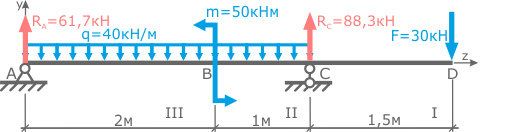To calculate the internal force factors along the sections of the beam, we use the section method.

Calculation of values

Draw a cross-section within the section, anywhere between points C and D

This section divides the beam into two parts (left and right). To determine internal factors, you can choose any of them, but it is better to choose a less loaded part of the beam. Obviously this will be its right side.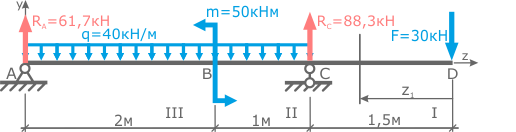The distance from the right boundary of the section to the section under consideration is denoted by the variable z1, which can take values ​​from 0 to 1.5 meters (i.e., 0 ≤ z1 ≤ 1.5 m).

Video by subject (contains English subtitles)

Mentally, we will discard the entire left side of the beam for a while.The transverse force Q in the given section of the first section will be equal to the sum of all external forces applied to the considered part of the beam, taking into account their sign, i.e.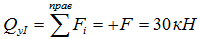Here, the force F is written as positive; tends to rotate the right side of the beam along the clockwise direction relative to the cross-section in question.

In this expression there is no variable z1, which means that the internal transverse force will be the same for all sections of this section.

The bending moment M in the considered section is defined as the sum of the bending moments from all external loads of the selected part of the beam.

Taking into account the rule of signs in bending, we obtain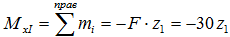Here the force F by the rule of signs is written negative, because strive to compress the lower layer of the beam.

In the expression obtained, the variable z1 is the arm of the moment of force F for a given beam section.

As can be seen from the expression obtained, the bending moment along the length of the section varies linearly (because z1 is in the first degree), therefore, for the plotting of the diagram on this section, two points are sufficient.

These points are the values ​​of the bending moment at the boundaries of the I section, i.e. at z1 = 0 and at z1 = 1,5 m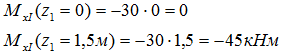In the first section, internal efforts are identified.

We pass to the second power section (BC).

Just start with the fact that we conduct a section in any place of the site and choose the part of the beam in question. It is also more convenient to consider the right side of the beam.

The distance to the considered section from the right boundary of the section is denoted by z2. In this case 0 ≤ z2 ≤ 1m.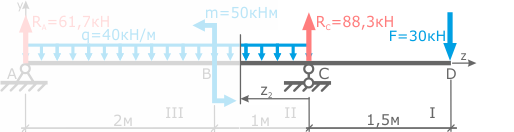We write the expressions and calculate the boundary values ​​of the internal transverse force QAnd the bending moment M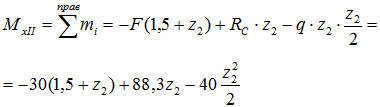Here, the RC reference is positive, because it compresses the upper layer, and the force F and the distributed load q are negative, because compress the lower layer of the beam.
How to record the moment of distributed load is shown here.

In the expression for MxII, the variable is in the second degree, therefore the moment diagram in the second section will have the form of a parabola.

As is well known, in order to construct a parabola, it is necessary to know the position of at least three of its points. But as will be shown later, in some cases when constructing the diagrams, the parabola can be drawn with only two points. We calculate their values: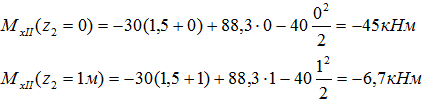It remains to find internal efforts on the third power section (AB)

We cut the beam between the points A and B. We choose the less loaded left part. 0 ≤ z3 ≤ 2m — the interval of possible positions of the section relative to the left boundary of the section.

Write the expressions for Q and M and calculate the values ​​at the end pointsIt is seen that the expression for QyIII is linear, and on the diagram Mx in this section there will be a parabola.

Based on the data obtained, we construct the diagrams.

Constructing diagrams

For the construction of the diagrams, we calculate the calculated values ​​from the baseline in the corresponding sections.

We begin with the diagrams of the transverse forces Q.

In the first section, the expression for Q does not depend on z1, so its value will be constant (QyI = const) along the length of the section, i.e. The line of the diagram will be parallel to the base.

In the second section, two values ​​of Q were obtained: -58.3 kN at z2 = 0 and -18.3 kN at z2 = 1 m. Variable z2 was plotted from the right boundary of the section, so z2 = 0 at point C, respectively in point B variable z2 = 1m.Similarly, the values ​​of Q in the third section and the values ​​of M on the bending moment diagram are plotted.

The points on the II and III sections of the diagram Q and on the I section of the diagram M are connected by segments, since the distribution of internal forces and moments there is linear (the variable z in the first degree).And when connecting the points of the diagram M with parabolas, one must look at the diagram Q.

The fact is that the transverse force diagram is the first derivative of the bending moment diagram. Therefore, in the cross sections of the beam, where Q = 0 on the diagram M, there will be an extremum.

As can be seen, the diagram Q intersects the zero line only in the third power section of the beam. Therefore, in view of the fact that we are only interested in the peak values ​​of the bending moments, in the second section, the two extreme points must be joined by a parabola that does not have an extremum whose convexity is directed toward the distributed load.

For a more accurate construction of the parabola line in this section, it is possible to find the moment values ​​for the intermediate positions of the section, for example, at z2 = 0.5 m.In the third section, in the section where Q crosses the baseline, it is necessary to calculate the extremum point.

To do this, the expression for QyIII equals zero and the value of z3 is calculated, at which the bending moment in the section takes an extreme value. It is substituted into the expression for MxIII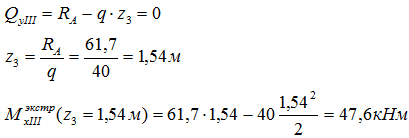This value is plotted on the diagram M below the intersection point of the Q diagram with the base lineafter which the three points are connected by a smooth line.The diagrams of the internal transverse forces and bending moments are constructed.